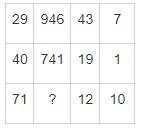Courses

# In the following question, select the number which can be placed at the sign of question mark (?) from the given alternatives.a)-49b)5.08c)-732d)732Correct answer is option 'D'. Can you explain this answer? Related Test: SSC CHSL Mock Test -8

## SSC QuestionLearners World Mar 05, 2021
Here the pattern followed in each row is:
In row 1 → 29 - (946 ÷ 43) = 29 - 22 = 7
In row 2 → 40 - (741 ÷ 19) = 40- 39 = 1
Similarly,
In row 3 → 71 - (? ÷ 12) = 10
→ -(? ÷ 12) = 10 – 71 = -61
→ ? = 61 × 12 = 732
Hence, 732 is the correct answer.

This discussion on In the following question, select the number which can be placed at the sign of question mark (?) from the given alternatives.a)-49b)5.08c)-732d)732Correct answer is option 'D'. Can you explain this answer? is done on EduRev Study Group by SSC Students. The Questions and Answers of In the following question, select the number which can be placed at the sign of question mark (?) from the given alternatives.a)-49b)5.08c)-732d)732Correct answer is option 'D'. Can you explain this answer? are solved by group of students and teacher of SSC, which is also the largest student community of SSC. If the answer is not available please wait for a while and a community member will probably answer this soon. You can study other questions, MCQs, videos and tests for SSC on EduRev and even discuss your questions like In the following question, select the number which can be placed at the sign of question mark (?) from the given alternatives.a)-49b)5.08c)-732d)732Correct answer is option 'D'. Can you explain this answer? over here on EduRev! Apart from being the largest SSC community, EduRev has the largest solved Question bank for SSC.